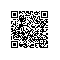# 算法设计与分析复习——第三章：动态规划

第三章：动态规划

1  分治算法和动态规划算法都是通过对问题进行分解，通过对子问题的求解然后进行解重构，从而实现对原问题的求解。请指出这两种算法在对问题进行分解时各自所遵循的原则。

2  动态规划算法的本质是什么，请简要阐述。

3  如果一个问题可以利用动态规划算法求解，那么该问题应满足什么条件？

4、动态规划算法的基本思想是什么？请简述动态规划算法主要设计步骤。

1）找出最优解的性质，并刻画其结构特征；

2）递归地定义最优值；

3）以自底向上的方式计算出最优值；

4）根据计算最优值时得到的信息，构造最优解；

5，什么是备忘录方法？它同动态规划法相比主要不同点是什么？请指出动态规划法和备忘录方法各自的适用范围。(注：平常说的“动态规划”是自底向上的动态规划，“备忘录方法”是自顶向下的动态规划。)

6，矩阵相乘算法目前最好的时间复杂度是多少？

7，分治法与动态规划法之间的相同点是什么？不同之处在哪些方面？

1，2，3，动态0-1背包

public class  SF_Beibao_0_1{

public static void main(String[]args){

int []v={1,2,3,4,5,6,7};

int []w={2,3,4,5,34,23,2};

int []b={2,3,4,1,2,3,5};

int c=45;

int d=36;

int [][][]m=new int[v.length][c+1][d+1];

knapsack(v,w,b,c,d,m);

System.out.println("输出最优解："+m[c][d]);

}

public static void knapsack(int[]v,int[]w,int []b,int c,int d,int[][][]m){

int n=v.length-1;

int jMax=Math.min(w[n]-1,c);

int kMax=Math.min(b[n]-1,d);

for(int j=0;j<=jMax;j++)

for(int k=0;k<=kMax;k++)

m[n][j][k]=0;

for(int j=w[n];j<=c;j++)

for(int k=b[n];k<=d;k++)

m[n][j][k]=v[n];

for(int i=n-1;i>1;i--){

jMax=Math.min(w[i]-1,c);

kMax=Math.min(b[i]-1,d);

for(int j=0;j<=jMax;j++)

for(int k=0;k<=kMax;k++)

m[i][j][k]=m[i+1][j][k];

for(int j=w[i];j<=c;j++)

for(int k=b[i];k<=d;k++)

m[i][j][k]=Math.max(m[i+1][j][k],m[i+1][j-w[i]][k-b[i]]+v[i]);

}

m[c][d]=m[c][d];

if(c>=w&&d>=b)m[c][d]=Math.max(m[c][d],m[c-w][d-b]+v);

}

public static void trackback(int[][][]m,int[]b,int[]w,int c,int d,int[]x){

int n=w.length-1;

for(int i=1;i<n;i++)

if(m[i][c][d]==m[i+1][c][d])x[i]=0;

else {x[i]=1;c-=w[i];d-=b[i];}

x[n]=(m[n][c][d]>0)?1:0;

}

}

4，使用钉钉扫一扫加入圈子
+ 订阅